How Cheenta works to ensure student success?
Explore the Back-Story

# Test of Mathematics Solution Subjective 48 - The Gifts DistributionThis is a Test of Mathematics Solution Subjective 48 (from ISI Entrance). The book, Test of Mathematics at 10+2 Level is Published by East West Press. This problem book is indispensable for the preparation of I.S.I. B.Stat and B.Math Entrance.

Also visit: I.S.I. & C.M.I. Entrance Course of Cheenta

## Problem

Find the different number of ways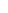different gifts can be presented to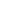children so that each child receives at least one gift.

## Solution:

There are two possible ways in which the gifts can be distributed.

Case 1: They are distributed as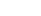.

So first we choose the children who get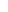gifts each in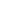ways. Then we choose the gifts in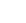ways.

Thus total number of ways =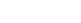ways.

Case 2: They are distributed as.

So first we choose the child who getsgifts  in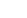ways. Then we choose the gifts in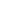ways.

Thus total number of ways =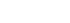ways.

Therefore total number of ways to distribute the gifts =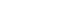=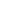ways.This is a Test of Mathematics Solution Subjective 48 (from ISI Entrance). The book, Test of Mathematics at 10+2 Level is Published by East West Press. This problem book is indispensable for the preparation of I.S.I. B.Stat and B.Math Entrance.

Also visit: I.S.I. & C.M.I. Entrance Course of Cheenta

## Problem

Find the different number of waysdifferent gifts can be presented tochildren so that each child receives at least one gift.

## Solution:

There are two possible ways in which the gifts can be distributed.

Case 1: They are distributed as.

So first we choose the children who getgifts each inways. Then we choose the gifts inways.

Thus total number of ways =ways.

Case 2: They are distributed as.

So first we choose the child who getsgifts  inways. Then we choose the gifts inways.

Thus total number of ways =ways.

Therefore total number of ways to distribute the gifts ==ways.

### Knowledge Partner